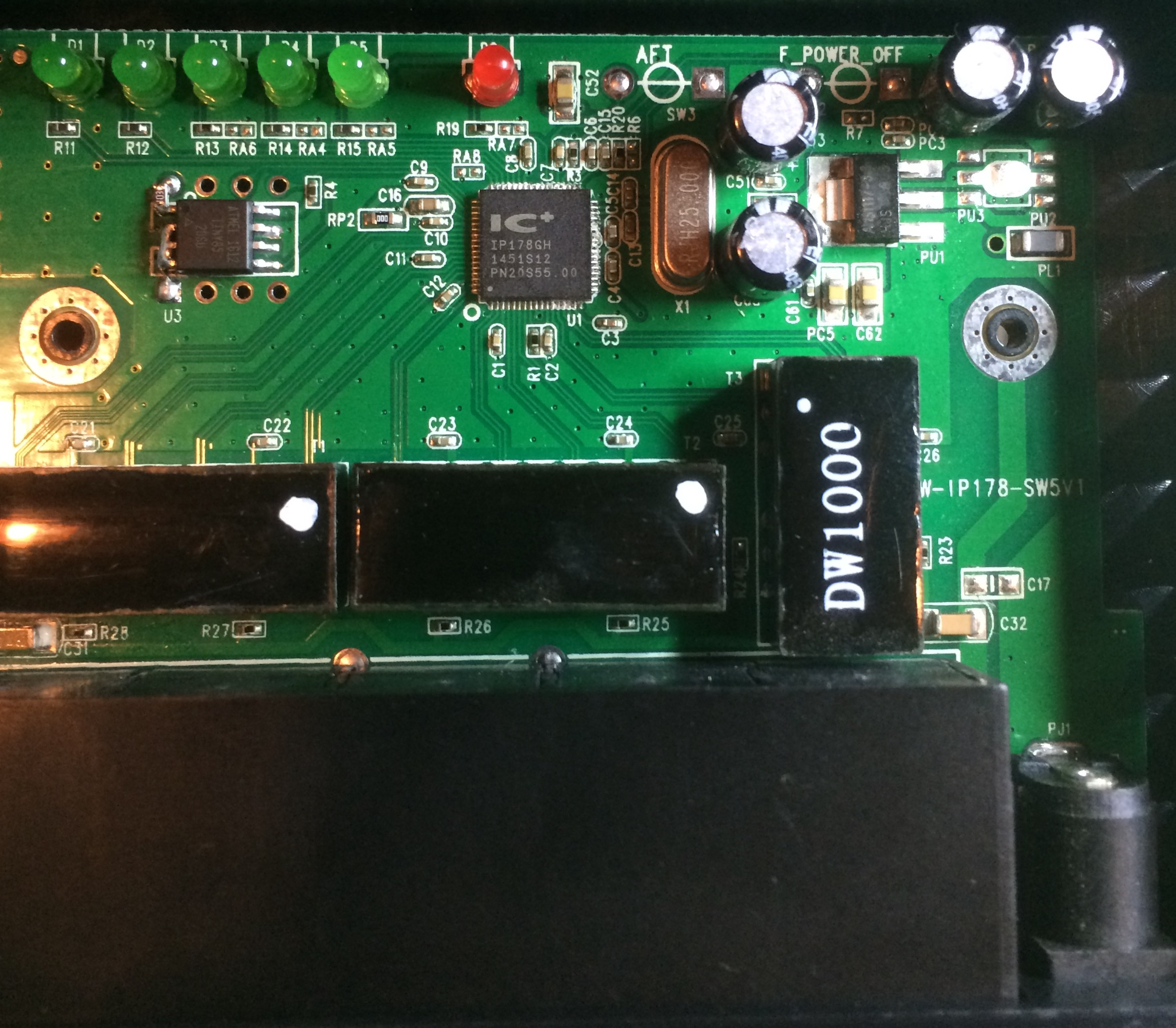## Creating the miror swtichSince I last wrote about adding management features to a cheap 5 port switch I’ve been trying to productionize that work.

I wanted to load the switch with a static config, enabling port mirroring from ports 0 to 1 as a network diagnostics tool (plug: you can now buy this on my shop).

The ip178g can load its config from flash at boot, and the flash IC footprint is on the board. Great! Should be easily… well turns out not so much. The flash used is an AT24C01, this was superseded by the AT24C01A and is no longer available from the normal suppliers. I had a lot of fun figuring this out (and no they’re not compatible).

Ordering the part from Shenzhen would take a month, and because the AT24C01A is also thrown around prety freely I’m not confident that I’d get the right part anyway.

So, I decided to use an ATTINY85. Actually at first I decided to use a PIC12F629 (this was a horror story in itself). I purchased some digisparks and used code adapted from my previous post to send config messages to the IP178G. It would also be possible to simulate the the AT24C01, which might be an interesting project.

Before I gave up on the AT24C01 route I wrote a flash image builder. You can find this can the digispark code on github.

To mount the ATTINY85 in the switch I pulled it off the digispark and placed it on the AT24C01 footprint. For the most part the pins line up acceptably well. The ATTINY85 is a wider part, and a pullup resistor needs to be added to the reset pin, but this is an acceptable solution for now (the rework is shown above).

Digispark code is below for reference:

```int clock_pin = 1;
int data_pin  = 0;

int width=100;

void setup() {
pinMode(clock_pin, OUTPUT); // CLOCK
pinMode( data_pin, OUTPUT); // DATA
}

int recv_one_bit() {
int data=0;
digitalWrite(clock_pin,0);
delayMicroseconds(width);
digitalWrite(clock_pin,1);
delayMicroseconds(width/2);
data = digitalRead(data_pin);
delayMicroseconds(width/2);
digitalWrite(clock_pin,0);
return data;
}

void send_data_bit(int data) {
digitalWrite(clock_pin,0);
delayMicroseconds(width/2);
digitalWrite(data_pin,data);
delayMicroseconds(width/2);
digitalWrite(clock_pin,1);
delayMicroseconds(width);
digitalWrite(clock_pin,0);
}

void outhigh() {
pinMode(data_pin,OUTPUT);
digitalWrite(data_pin,1);
digitalWrite(clock_pin,0);
}

void send_data(uint32_t data,int len) {

for(int32_t n=len-1;n>=0;n--) {
if(data & ((uint32_t)1 << n)) { send_data_bit(1); } else { send_data_bit(0); }
}
}

uint32_t recv_data(int len) { uint32_t datain = 0; for(int32_t n=(len-1);n>=0;n--) {
int d = recv_one_bit();
if(d != 0) {
datain = datain | (uint32_t)((uint32_t)1 << n);
}
}
return datain;
}

uint32_t read_op(int phy,int reg) {

pinMode(data_pin,OUTPUT);
send_data_bit(1);

send_data_bit(0); // start bits
send_data_bit(1);
send_data_bit(1); // read operation
send_data_bit(0);

send_data(phy,5);
send_data(reg,5);

pinMode(data_pin,INPUT);

uint32_t in;
int inA = recv_one_bit(); // turn around
//int inB = recv_one_bit(); // this should be required as per spec, but a bit gets missed if I use it.

in = recv_data(16);

outhigh();

return in;
}

void write_op(int phy,int reg,uint32_t data) {

pinMode(data_pin,OUTPUT);
send_data_bit(1);

send_data_bit(0);
send_data_bit(1);
send_data_bit(0);
send_data_bit(1);

send_data(phy,5);
send_data(reg,5);

send_data_bit(1);
send_data_bit(0);
//  send_data(2,2); // Turnaround - required by spec, but causes issues.

send_data(data,16);
}

void loop() {

outhigh();
delay(5000); // one second

//Port mirroring config sits in phy 20, regs 3 and 4.
//The bit pattern is shown below. See the datasheet for further details.
//15.......................0
//   enable, mode, res, mirrored port rx
//3: 1,   11,00000,00000001
//
//   monitoring port, res, mirrored port tx
//4: 001 ,00000,00000001

uint32_t reg3 = 0xE001;
uint32_t reg4 = 0x2001;

write_op(20,3,reg3);
write_op(20,4,reg4);
delay(1000*30); // 30s
}
```